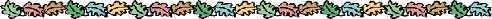# Geometry### 3-D Geometry & Polyhedra

Math Forum's Studying Polyhedra
Interactive: Study the Platonic solids.
Paper Models of Polyhedra
80+ nets of polyhedral models.
MatHSoliD Java Applet (Interactive)
All Platonic and Archimedean Solids.
NCTM's Illuminations (Interactive)
Geometric Solids and Their Properties: Grades 3-5.
NCTM's Illuminations (Interactive)
Cubes and Isometric Drawings: Students
NCTM's Illuminations (Interactive)
Cubes and Isometric Drawings: Teachers
The Geometry Junkyard: Unfolded Polyhedra
Pedagoguery Software Inc. (Interactive)
Math Cats: Geometric Space Forms
Create polyhedral nets with compass and straightedge
Straw Polyhedra
Soda staws & string.
Virtual Polyhedra - An Encyclopedia of Polyhedra
Thousands of virtual reality polyhedra to explore.
University of Utah
Interactive Platonic Solids via Java applet.

### Constructions

Kocmoud's Constructions WIMS Ruler & Compass Constructions MathsNet Interactive Constructions
Grand Valley State: Geometric Constructions

### Geometry of Nature

Book: The Geometry of Nature Pictures: The Geometry of Nature

### M.C. Escher

MCEscher.com
Escher's official Website.
The Oldest Escher Collection of the Web
Works by Escher & artists inspired by him.
MC Escher - Artist or Mathematician ?
Biographical detail and tutorial.

### Similar Polygons

Pink Monkey Notes - Similar Polygons

### Tessellations

Math Formum Tessellation Tutorial
Definitions, samples, interactive tessellation engine.
Tantalizing Tessellations
Ten complete tessellation lessons.
David Annal's Tessellations.org
History, gallery, do-it-yourself.
Science U's Introduction to Tilings and Tessellations
Examples and 2 interactive tessellation engines.
Cool Math's Tessellation Page
Definitions and examples.

### Transformational Geometry & Symmetry

MathsNet Transformational Geometry Unit
Interactive unit: four levels of instruction.

Leslie Howe's Transformation Unit
Simple interactive unit.
NCTM: Congruence, Similarity, and Symmetry
Visualizing transformations: interactive applets.
UGA's Transformational Geometry Unit
Ten day unit for Geometer's SketchPad.
Java Gallery of Interactive Geometry Gallery of Interactive Geometry

### Pick's Theorem

Cut The Knot (Interactive)
Applet to demonstrate Pick's Theorem.
IES Manipula Math Java Applets
279 math applets. 91 in middle school geometry

How to Choose a Real-World Problem
Geometry Learning Units
This site provides a variety of geometry lessons that use real-world problem scenarios.
How Now Pythagoras?
This site provides a fun activity using the Pythagorean theorem for students interested in woodworking.
Determining Altitude of Iridium Flares
This site provides an activity for students interested in space exploration. Note this activity is more advanced.
The Goal Angle Problem
This site provides a fun activity exploring angles for students interested in soccer.
Geometry Through Art
This site uses art as a means for learning geometer’s sketchpad.
Lesson Plans Using GSP
This site provides objectives and lesson plans for grades 6-8. These lessons are for use with the Geometer’s Sketchpad.
Lesson Plans for the Geometer’s Sketchpad
This site provides 17 lesson plans that use the Geometer’s Sketchpad.
GSP Lessons
This site provides 13 lesson plans written for use with the Geometer’s Sketchpad.
The Geometer’s Sketchpad Equations of Circles
This site provides a GSP lesson plan for generating the equation of circles.